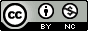## Wednesday, November 17, 2010

### Syntax Highlighting R Code, Revisited

A few months ago I showed you how to syntax-highlight R code using Github Gists for displaying R code on your blog or other online medium. The idea's really simple if you use blogger - head over to gist.github.com, paste in your R code, create a public "gist", hit "embed", then copy the javascript onto your blog. However, if you use a hosted wordpress.com or other blogging platform that doesn't allow javascript tags within a post, you can't use this method.

While I still prefer using Github Gists for archiving and version control, there's an alternative that works where javascript doesn't. Inside-R has a nice tool - Pretty R Syntax Highlighter - where you simply paste in your R code, and it generates HTML code that syntax-highlights your R code. What's more, functions in your R code link back to the documentation on inside-R.org. Here's an example of some code I posted a while back on making QQ plots of p-values using R base graphics.

Without any highlighting, it's hard to read, and spacing isn't faithfully preserved:

# Define the function
ggd.qqplot = function(pvector, main=NULL, ...) {
o = -log10(sort(pvector,decreasing=F))
e = -log10( 1:length(o)/length(o) )
plot(e,o,pch=19,cex=1, main=main, ...,
xlab=expression(Expected~~-log(italic(p))),
ylab=expression(Observed~~-log(italic(p))),
xlim=c(0,max(e)), ylim=c(0,max(o)))
lines(e,e,col="red")
}

#Generate some fake data that deviates from the null
set.seed(42)
pvalues=runif(10000)
pvalues[sample(10000,10)]=pvalues[sample(10000,10)]/5000

# pvalues is a numeric vector
pvalues[1:10]

# Using the ggd.qqplot() function
ggd.qqplot(pvalues)

ggd.qqplot(pvalues, "QQ-plot of p-values using ggd.qqplot")

.......
Here's the same code embeded using Github Gists:

And the same code using Inside-R.org's Pretty R Syntax Highlighter. Note that function calls are hyperlinks to the function's documentation on inside-R.

```# Originally posted at http://gettinggeneticsdone.blogspot.com/2010/07/qq-plots-of-p-values-in-r-using-base.html

# Define the function
ggd.qqplot = function(pvector, main=NULL, ...) {
o = -log10(sort(pvector,decreasing=F))
e = -log10( 1:length(o)/length(o) )
plot(e,o,pch=19,cex=1, main=main, ...,
xlab=expression(Expected~~-log(italic(p))),
ylab=expression(Observed~~-log(italic(p))),
xlim=c(0,max(e)), ylim=c(0,max(o)))
lines(e,e,col="red")
}

#Generate some fake data that deviates from the null
set.seed(42)
pvalues=runif(10000)
pvalues[sample(10000,10)]=pvalues[sample(10000,10)]/5000

# pvalues is a numeric vector
pvalues[1:10]

# Using the ggd.qqplot() function
ggd.qqplot(pvalues)

ggd.qqplot(pvalues, "QQ-plot of p-values using ggd.qqplot")```

Have any other solutions for syntax highlighting R code? Please share in the comments!

Github Gists
Inside-R Pretty R Syntax Highlighter

1.RKward exports syntax highlighted HTML of R code as well.

Granted, it creates a whole page, but if you just copy the code between the < body> tags you can copy/paste into a blog.

2.I wrote my tips for WordPress blogs here:
http://www.r-statistics.com/2010/10/wp-codebox-a-better-r-syntax-highlighter-plugin-for-wordpress/